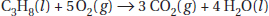# AP Chemistry Practice Test 11

### Test Information12 questions18 minutes

1. What is the equilibrium expression for the reaction2. The rate law may be written using stoichiometric coefficients for which of the following?

3. A certain radioactive material has a half-life of 36 minutes. Starting with 10.00 grams of this material, how many grams will be left after 2 hours?

4. A solution is prepared by dissolving 30.0 grams of Ni(NO3)2 in enough water to make 250 mL of solution. What is the molarity of this solution?

5. Hard materials such as silicon carbide, used for grinding wheels, are said to be examples of

6. The equilibrium constant, Kc, for the dissociation of HI into hydrogen gas and iodine vapor is 21 at a certain temperature. What will be the molar concentration of iodine vapor if 15 grams of HI gas is introduced into a 12.0-L flask and allowed to come to equilibrium?

7. What is the molarity of a sodium hydroxide solution that requires 42.6 mL of 0.108 M HCl to neutralize 40.0 mL of the base?

8. Changing which of the following will change the numerical value of the equilibrium constant?

9. The simplest alkene has

10. Helium effuses through a pinhole 5.33 times faster than an unknown gas. That gas is most likely

11. The Pauli exclusion principle states that

12. Which of the following can form hydrogen bonds?